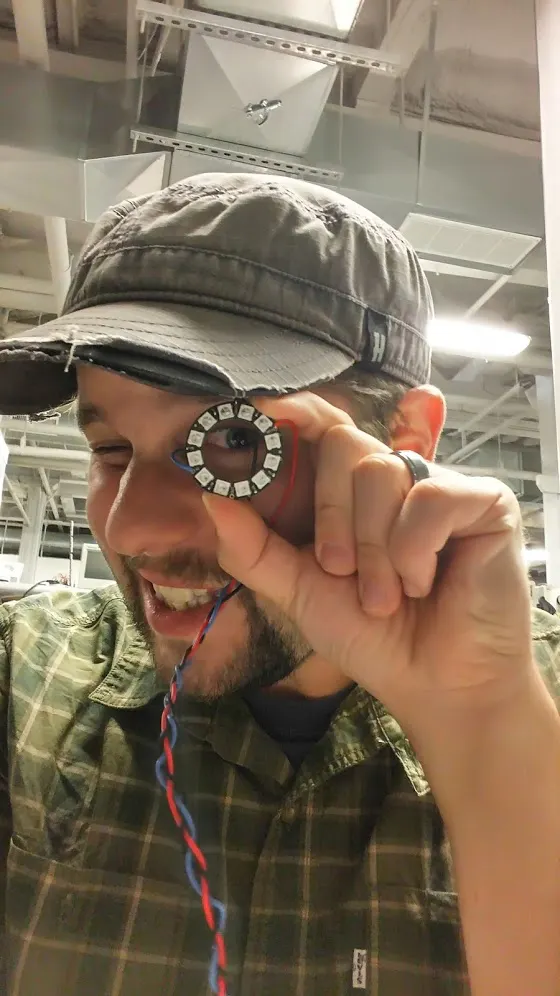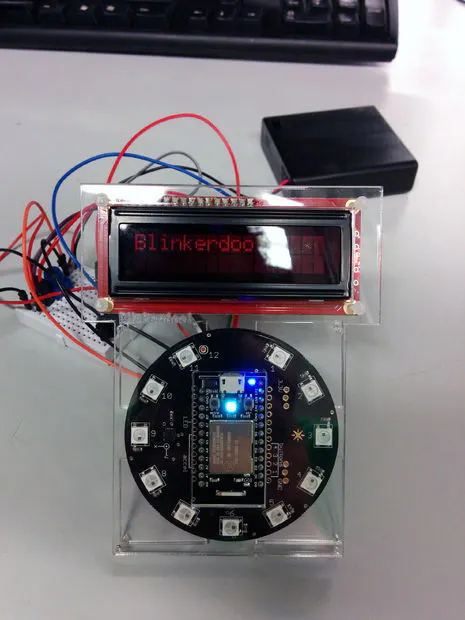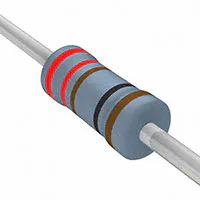Published

Single player reaction light game that also tells the weather

Full instructions provided795## Things used in this project

### Hardware components

 Spark Core microcontroller
×1
 the Sparkbutton
×1
 3.3 V LCD screen
×1Resistor 221 ohm
×1
 female to male jumper wires
×12
 Male to Male Jumper Wires
×4
 Potentiometer
×1×1

## Code

### file_9569.txt

C/C++
```//import statements for libraries will be automatically included by the sparkCore IDE when copied

//this module is to create a concept game using the sparkbutton to power LEDs on the ring and interact with them using two of the buttons on the board

//it also has an additional "weather mode" that is called using the third/bottom button on the sparkbutton

Weather* weather; //activate openweather, include everything

HttpClient* httpClient; //activate HttpClient, include everything

SparkButton b = SparkButton();//claim button object

LiquidCrystal lcd(0, 5, 6, 7, A0, A1); //assign pin outs to LCD screen

void setup()

{

lcd.begin(16, 2); //LCD screen will be 16 x 2 characters

Serial.begin(9600); //serial USB

b.begin();//activate button

httpClient = new HttpClient(); //new HttpClient object

//weather->setCelsius();

weather->setFahrenheit();

}

void loop()

{

lcd.display(); //turn on LCD

delay(100); //delay 100 ms

lcd.clear(); //clear LCD

if (b.buttonOn(3)) //if button 3 is pressed, go into weathermode

{

lcd.clear();

weatherMode(); //call weather function

lcdWeather(); //call lcd function

} //end if

else //otherwise game mode

{

gameMode(); //call game function

} //end else

} // end loop

void gameMode() //function for game mode

{

bool led3 = true; //boolean variables to dictate behavior of lights and interaction

bool led9 = false; // with buttons

int n; //light for button 2

int m; //light for button 4

int t = 100; //time constant

for(t = 100; t > 5; t--) //speed of light hastens with every cycle

{

if(b.buttonOn(2) && led3 == true) //if button 2 is pressed LEDs bounce from 3 to 9

{

for (n=3; n <= 9; n++)

{

b.ledOn(n, 0,0,255); //blue led travels

delay(t);

b.ledOff(n-1);

delay(t);

led9 = true;

}

}

else if(b.buttonOn(4) && led9 == true)//if button 4 is pressed LEDs bounce from 9 to 3

{

for (m = 9; m >= 3; m--)

{

b.ledOn(m, 0,255,0); //green led travels back

delay(t);

b.ledOff(m+1);

delay(t);

led9 = false;

}

}

else //if player fails to complete cycle, game is over.

{

b.allLedsOff();

break;

}

}

}

void weatherMode() //weather function

{

weather_response_t resp = weather->cachedUpdate(); //ping weather API

if (resp.isSuccess) //if ping is successful give read out

{

Serial.print(resp.temp_low);

Serial.print(" - ");

Serial.print(resp.temp_high);

Serial.print(" - ");

Serial.println(resp.descr);

delay(100);

int t = resp.temp_high;        //if ping is successful, change LEDs on button according to high temperature for the day, can also use case statements

if (t > 90)

{

b.allLedsOn(55,0,0); //if temperature is above 90, red LEDs

}

else if ((t < 90) && (t >= 80))

{

b.allLedsOn(255,128,0); //if temperature is between 90 and 80, orange LEDs

}

else if ((t < 80) && (t >= 70 ))

{

b.allLedsOn(55,55,0); //if temperature is between 80 and 70, yellow LEDS

}

else if ((t < 70) && (t >= 60))

{

b.allLedsOn(0, 55, 0); //if temperature is between 70 and 60, green LEDs

}

else if ((t < 60) && (t >= 50))

{

b.allLedsOn(0, 0, 55); //if temperature is between 60 and 50, blue LEDs

}

else if((t < 50) && (t >= 32))

{

b.allLedsOn(55, 0, 55); //if temperature is between 40 and freezing, purple LEDs

}

else

{

b.allLedsOn(50, 50, 50); //if temperature is below freezing, white LEDs

}

}

} //end weatherMode()

void lcdWeather() //lcd readout of weather

{

lcd.display(); //turn on display

weather_response_t resp = weather->cachedUpdate();

if (resp.isSuccess) //if ping is successful, give readout on LCD

{

lcd.print(resp.temp_low); //gives low temp for day

lcd.print(" - ");

lcd.print(resp.temp_high); //gives high temp for day

lcd.setCursor(0,1);       //returns

lcd.print(resp.descr); //gives condition for the day

delay(3500);

lcd.clear(); //clears LCD after 3.5 seconds

} //end if

} //end lcdWeather function
```

## Credits

### Jonathan Eskow

5 projects • 17 followers
Electronics Engineer at NextFab and ECE undergrad at Temple University